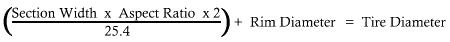Metric Tire to Inches enter 3 of 4 in green, press CALC Example: LT235/75R16 = 30" LT / R = Diameter '' Section Width:''   Section Height:''

METRIC TIRE TO DIAMETER (INCHES) CALCULATOR

This calc converts a metric tire to inches.
Solve for Section Width (P or LT).
Solve for Aspect Ratio (width).
Solve for Rim Diameter (inches).
Solve for Tire Diameter (inches).
Solve for Section Width/Height (inches).

Most of my calculators will ask for your tire diameter, if you have metric tires (most of us do) you'll need to get an estimate of how tall they are. Or you can just pull out the ole tape measure.

Most of the formulas dealing with gear ratios will want a tire diameter (measured in inches). This formula is a quick way to get the tire diameter of those metric tires that are common on just about everything stock. For example a LT265/75R16 would be around 31.6 inches tall and 10 inches wide. Enter any three of the numbers into this form to solve for the fourth. "LT" means Light Truck and "P" means Passenger tire. The bigger number (on the left) is the Section Width. The number to the right of the slash ("/") is the Aspect Ratio (percent of width). The "R" means Radial tire and the last number, far right, is the rim diameter (in inches!).

Formula usedWidth in inches = section width / 25.4

Section Height in inches = Width in inches X Aspect Ratio (%)

 These calculators are color coded by function All fields Required Leave ONE field blank Supply ONLY one field Results Only